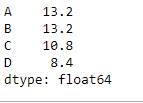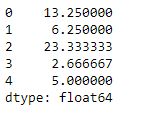# Pandas DataFrame mean() Method

Python is a great language for doing data analysis, primarily because of the fantastic ecosystem of data-centric Python packages. Pandas is one of those packages and makes importing and analyzing data much easier.

## Pandas DataFrame mean()

Pandas dataframe.mean() function returns the mean of the values for the requested axis. If the method is applied on a pandas series object, then the method returns a scalar value which is the mean value of all the observations in the Pandas Dataframe. If the method is applied on a Pandas Dataframe object, then the method returns a Pandas series object which contains the mean of the values over the specified axis.

Syntax: DataFrame.mean(axis=None, skipna=None, level=None, numeric_only=None, **kwargs)

Parameters :

• axis : {index (0), columns (1)}
• skipna : Exclude NA/null values when computing the result
• level : If the axis is a MultiIndex (hierarchical), count along a particular level, collapsing into a Series
• numeric_only : Include only float, int, boolean columns. If None, will attempt to use everything, then use only numeric data. Not implemented for Series.

Returns : mean : Series or DataFrame (if level specified)

## Pandas DataFrame.mean() Examples

Example 1:

Use mean() function to find the mean of all the observations over the index axis.

## Python3

 `# importing pandas as pd ` `import` `pandas as pd ` ` `  `# Creating the dataframe ` `df ``=` `pd.DataFrame({``"A"``:[``12``, ``4``, ``5``, ``44``, ``1``], ` `                ``"B"``:[``5``, ``2``, ``54``, ``3``, ``2``], ` `                ``"C"``:[``20``, ``16``, ``7``, ``3``, ``8``], ` `                ``"D"``:[``14``, ``3``, ``17``, ``2``, ``6``]}) ` ` `  `# Print the dataframe ` `df `Let’s use the Dataframe.mean() function to find the mean over the index axis.

## Python3

 `# Even if we do not specify axis = 0, ` `# the method will return the mean over ` `# the index axis by default ` `df.mean(axis ``=` `0``) `

Output:Example 2:

Use mean() function on a Dataframe that has None values. Also, find the mean over the column axis.

## Python3

 `# importing pandas as pd ` `import` `pandas as pd ` ` `  `# Creating the dataframe ` `df ``=` `pd.DataFrame({``"A"``:[``12``, ``4``, ``5``, ``None``, ``1``], ` `                ``"B"``:[``7``, ``2``, ``54``, ``3``, ``None``], ` `                ``"C"``:[``20``, ``16``, ``11``, ``3``, ``8``],. ` `                ``"D"``:[``14``, ``3``, ``None``, ``2``, ``6``]}) ` ` `  `# skip the Na values while finding the mean ` `df.mean(axis ``=` `1``, skipna ``=` `True``) `

Output:Whether you're preparing for your first job interview or aiming to upskill in this ever-evolving tech landscape, GeeksforGeeks Courses are your key to success. We provide top-quality content at affordable prices, all geared towards accelerating your growth in a time-bound manner. Join the millions we've already empowered, and we're here to do the same for you. Don't miss out - check it out now!

Previous
Next